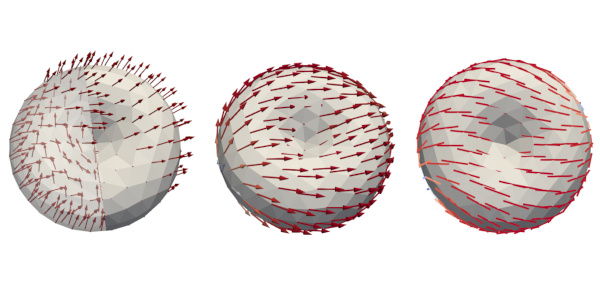## Symmetry, length, and tangential constraintsMonolayers of anisometric molecules on curved surfaces show a non-trivial orientational order depending on the shape and symmetry of the molecules but also on the topology and curvature of the domain. The geometry of the surface changes the behavior of the attached particles compared to a flat environment. Physical models represent the preferred microscopically averaged molecular orientation by vectorial or tensorial order parameters with additional constraints, like unit length and rotation symmetry. Length constraints can be phrased as an $\mathcal{S}^2$ target-manifold constraint, head-tail symmetry as an $\mathbb{R}P^2$ constraint, and rotational symmetry in terms of quotient spaces. In addition, molecules might be enforced to align tangentially to the surface. This gives an orientational tangentiality constraint that depends on the geometry of the domain. To simulate such a model with a combination of symmetry, length, and tangential constraints, numerical methods are required that handle the interaction between constraints and surface geometry. Each of the constraints as well as the geometry can either be encoded exactly in a hard manner or approximately in a soft manner. In this project, we investigate the influence of these design choices on finite element schemes and their convergence behavior. While each aspect of the model has been discussed separately in the literature before, a rigorous numerical analysis of finite element schemes for the interaction of multiple constraints and surface discretization has not been addressed. As this interaction influences both the qualitative structure of discrete solutions as well as their convergence behavior, our studies enable more reliable simulations compared to ad-hoc methods. Geometric finite elements have proven to be a successful method for the discretization of functions with hard Riemannian manifold constraints. The numerical analysis of these methods shows optimal convergence behavior and they are applied to various target manifolds. For handling functions in the setting of surface vector fields, the methods need to be extended in several directions. First of all, the surface domain is curved and triangulated, leading to questions regarding conformity and consistency of the method. Second, the codomain of the mappings might be tightly coupled to the domain, e.g., in the case of fiber bundle constraints. Third, the target manifolds in the above models do not always allow for a smooth structure which is required in the classical geometric finite element settings. We extend the finite element methods in all these directions, combine with soft enforcement of some of the constraints via penalization, and apply them to model problems from the context of liquid crystals.

### Publications

 Simon Praetorius and Florian Stenger, Dune-CurvedGrid – A Dune module for surface parametrization, In Archive of Numerical Software, Vol. 6 (1), pp. 1–27, 2022. [doi] [bibtex] Hanne Hardering and Simon Praetorius, Tangential Errors of Tensor Surface Finite Elements, In IMA Journal of Numerical Analysis, 2022, drac015. [doi] [bibtex]